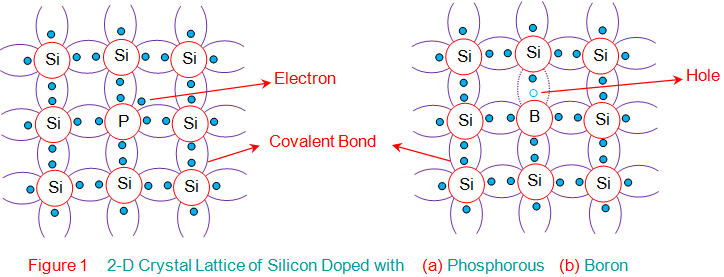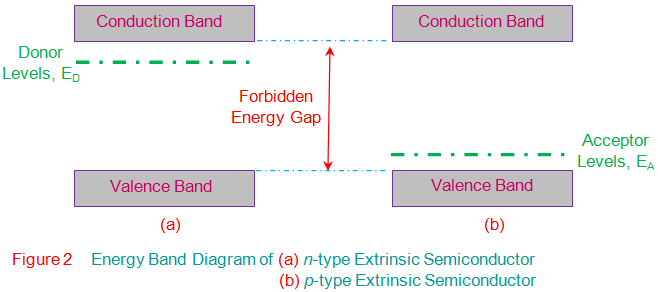# Extrinsic Semiconductors

Semiconductor materials can be classified into two types viz., Intrinsic Semiconductors and Extrinsic Semiconductors. Semiconductors in their pure form are referred to as intrinsic semiconductors. On the other hand, the semiconductors with intentionally added impurities are called extrinsic semiconductors. This process of adding impurities in minute quantities into the pure semiconductor material under controlled conditions is known as doping. The process is undertaken with the intention of increasing the conductivity of the material. Hence, the impurities are chosen in such a way that their addition into the pure semiconductor should increase the number of free charge carriers which can be either holes or electrons.

It is well known that the pure semiconductors like Silicon (Si) or Germanium (Ge) are tetravalent (have four electrons in their valence shell) as they belong to Group IV of the periodic table. Thus, if one needs to increase the number of electrons in them, they are to be doped with the Group V elements of the periodic table viz., Phosphorous (P), Arsenic (As), Antimony (Sb), Bismuth (Bi) or Lithium (Li). This is because Group V elements are pentavalent in nature, meaning which they have five electrons in their valence shell. This indicates that even after the formation of four covalent bonds due to the sharing of four electrons between the Group V and Group IV elements, one more electron (fifth electron of Group V element) would be left-behind as shown by Figure 1a.

In other words, pentavalent impurities are said to donate their electron to the tetravalent (pure) semiconductors and are hence called donors. The electron so donated will be very loosely bound to its parent atom and can thus be made ‘free’ by supplying very minimal amount of thermal energy. Such an excitation causes the electron to transit from its current energy state, donor level ED (green line in Figure 2a) to the conduction band. From then on it can actively contribute to the process of conduction in the semiconductor material along with the other free electrons generated due to the breaking of covalent bonds. From this, one can note that the total number of electrons in such a material is the sum of thermally generated electrons and the electrons donated by the donor atoms.

However, the number of holes in the same material remains equal to the number of thermally generated electrons only. This indicates that the number of electrons in this kind of material exceeds the number of holes, which means the electrons will be the majority charge carriers while the holes will be the minority carriers. Thus, these materials are aptly named as n type semiconductors.Similarly, the Group IV elements can also be doped with Group III elements of the periodic table like Boron (B), Aluminium (Al), Nitrogen (N), Gallium (Ga) or Indium (In). In this case, the resultant material will have three covalent bonds formed by the pairing-up of three electrons from each atom. However, due to the trivalent (three valence electrons) nature of Group III elements, there will be one insufficient electron to form the fourth covalent bond. This deficiency in electron signifies nothing but the hole as shown in Figure 1b.

The number of holes so formed is equal to the number of trivalent impurity atoms added into the semiconductor. Each of these holes signifies a vacant space which can readily accept an electron into it and is present at the energy level EA shown in Figure 2b. Further due to the nature of ‘acceptance’ exhibited by the holes induced due to the doping of Group III elements, such elements are referred to as acceptors.

Moreover, it is to be noted that, as an electron moves into the hole to recombine, it leaves a new hole in its previous place which in turn will be filled by some other electron. This indicates that the movement of electrons in one direction can be viewed as the movement of holes in the opposite direction. Thus, such a semiconductor material becomes conductive in nature. However, it is to be noted that, for this case, the total number of holes will be equal to the sum of the holes induced due to doping and the holes generated due to the thermal excitation process, unlike the electrons which are produced only due to the process of thermal excitation. This means that here the number of holes exceeds the number of electrons for which the holes will be the majority charge carriers while the electrons will be the minority charge carriers. Due to these kinds of extrinsic semiconductor materials are aptly called p type semiconductors.

Want To Learn Faster? 🎓
Get electrical articles delivered to your inbox every week.
No credit card required—it’s 100% free.